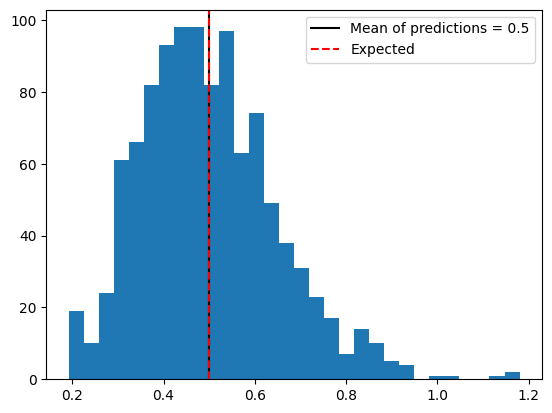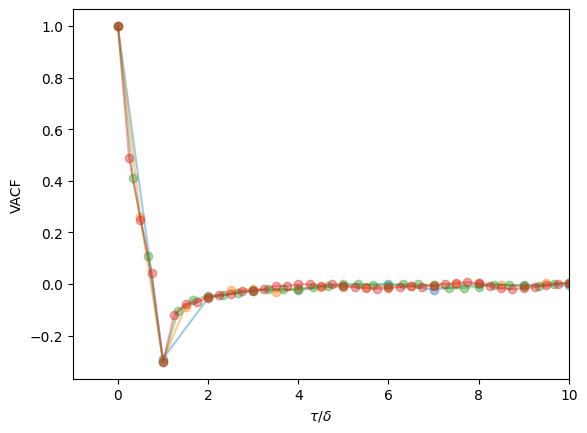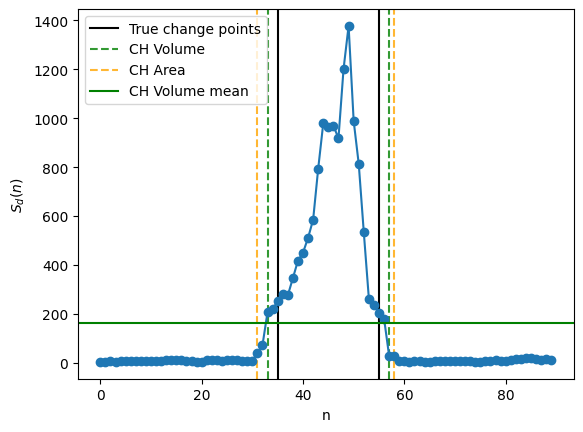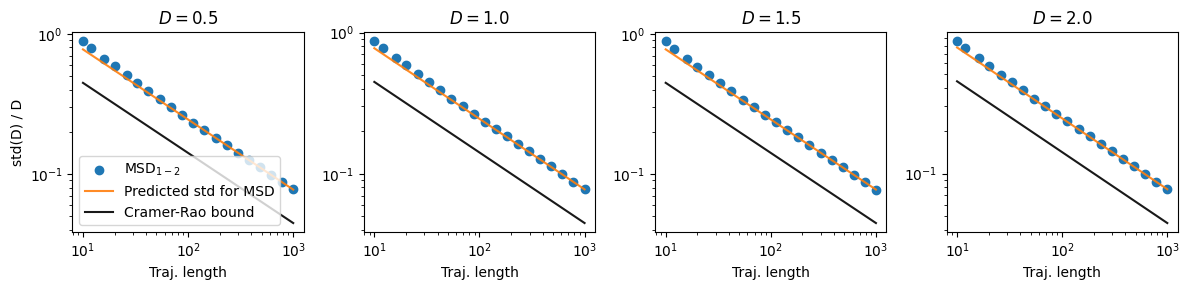# Angle calculation

source

### dataset_angles

 dataset_angles (trajs:list)

Given a set of trajectories, calculate all angles between displacements

Type Details
trajs list set of trajectories from which to calculate angles
Returns list list of angles between displacements

source

### get_angle

 get_angle (a:tuple, b:tuple, c:tuple)

Calculates the angle between the segments generate by three points

Type Details
a tuple 2d position point A
b tuple 2d position point B
c tuple 2d position point C
Returns tuple angle between segments AB and BC points

# MSD based analysis

source

### msd_analysis

 msd_analysis ()

Contains mean squared displacement (MSD) based methods to analyze trajectories.

source

### msd_analysis.tamsd

 msd_analysis.tamsd (traj:numpy.ndarray, t_lags:numpy.ndarray)

Calculates the time average mean squared displacement (TA-MSD) of a trajectory at various time lags,

Type Details
traj ndarray Trajectory from whicto calculate TA-MSD.
t_lags ndarray Time lags used for the TA-MSD
Returns np.array TA-MSD of the given trayectory

source

### msd_analysis.get_diff_coeff

 msd_analysis.get_diff_coeff (traj:numpy.ndarray, t_lags:list=None)

Calculates the diffusion coefficient of a trajectory by means of the linear fitting of the TA-MSD.

Type Default Details
traj ndarray 1D trajectory from whicto calculate TA-MSD.
t_lags list None Time lags used for the TA-MSD.
Returns np.array Diffusion coefficient of the given trajectory.

Here we show an example for the calculation of a Brownian motion trajectory. We create 100 trajectories from displacements of variance $$\sigma =1$$, which results in a diffusion coefficient $$D=0.5$$.

D = []
for _ in range(1000):
pos = np.cumsum(np.random.randn(100))
D.append(msd_analysis().get_diff_coeff(pos))

plt.hist(D, bins = 30);
plt.axvline(np.mean(D), c = 'k', label = f'Mean of predictions = {np.round(np.mean(D), 2)}')
plt.axvline(0.5, c = 'r', ls = '--', label = 'Expected')
plt.legend()
<matplotlib.legend.Legend>source

### msd_analysis.get_exponent

 msd_analysis.get_exponent (traj, t_lags:list=None)

Calculates the anolaous of a trajectory by means of the linear fitting of the logarithm of the TA-MSD.

Type Default Details
traj np.array 1D trajectory from whicto calculate TA-MSD.
t_lags list None Time lags used for the TA-MSD.
Returns np.array Anomalous exponent of the given trajectory.

To showcase this function, we generate fractional brownian motion trajectories with $$\alpha = 0.5$$ and calculate their exponent:

alpha = []
trajs, _ = models_phenom().single_state(N = 1000, T = 100, alphas = 0.5)
for traj in trajs.transpose(1,0,2):
alpha.append(msd_analysis().get_exponent(traj[:,0]))

plt.hist(alpha, bins = 30);
plt.axvline(np.mean(alpha), c = 'k', label = f'Mean of predictions = {np.round(np.mean(alpha), 2)}')
plt.axvline(0.5, c = 'r', ls = '--', label = 'Expected')
plt.legend()
<matplotlib.legend.Legend># Velocity Autocorrelation Function (VACF)

source

### vacf

 vacf (trajs, delta_t:int|list|numpy.ndarray=1,
taus:bool|list|numpy.ndarray=None)

Calculates the velocity autocorrelation function for the given set of trajectories.

Type Default Details
trajs np.array NxT matrix containing N trajectories of length T.
delta_t int | list | numpy.ndarray 1 If not None, the vacf is calculated in the demanded time lags.
taus bool | list | numpy.ndarray None Time windows at wich the vacf is calculated.
Returns np.array VACF of the given trajectories and the given time windows.

We show here an example of the VACF for FBM trajectories at various time lages, showing that they all coincide (as expected for this diffusion model).

deltats = np.arange(1, 5).tolist()
taus = np.arange(0, 100)
trajs, _ = models_phenom().single_state(N = 200, T = 200, alphas = 0.5)
trajs = trajs.transpose(1, 0, 2)[:,:,0]

for deltat in deltats:
v = vacf(trajs, deltat, taus)
plt.plot(taus/deltat, v.flatten(), 'o-', alpha = 0.4)
plt.xlim(-1, 10)
plt.ylabel('VACF'); plt.xlabel(r'$\tau / \delta$')
Text(0.5, 0, '$\\tau / \\delta$')# Convex hull analysis

source

### CH_changepoints

 CH_changepoints (trajs, tau:int=10, metric:{'volume','area'}='volume')

Computes the changes points a multistate trajectory based on the Convex Hull approach proposed in PRE 96 (022144), 2017.

Type Default Details
trajs np.array NxT matrix containing N trajectories of length T.
tau int 10 Time window over which the CH is calculated.
metric {‘volume’, ‘area’} volume Calculate change points w.r.t. area or volume of CH.
Returns list Change points of the given trajectory.

We showcase the use of the convex hull in a Brownian motion trajectory with two distinct diffusion coefficients, one 10 times the other:

# Generate trajectories and plot change points
T = 100; on = 40; off = 60;tau = 5
traj = np.random.randn(T, 2)
traj[on:off, :] = traj[on:off, :]*10
traj = traj.cumsum(0)
plt.axvline(on-tau, c = 'k')
plt.axvline(off-tau, c = 'k', label = 'True change points')

# Calculate variable Sd
Sd = np.zeros(traj.shape-2*tau)
for k in range(traj.shape-2*tau):
Sd[k] = ConvexHull(traj[k:(k+2*tau)], ).volume

# Compute change points both with volume and area
CPs = CH_changepoints([traj], tau = tau).flatten()-tau
CPs_a = CH_changepoints([traj], tau = tau, metric = 'area').flatten()-tau

# Plot everything
label_cp = 'CH Volume'
for cp in CPs:
plt.axvline(cp, c = 'g', alpha = 0.8, ls = '--', label = label_cp)
label_cp = ''
label_cp = 'CH Area'
for cp in CPs_a:
plt.axvline(cp, alpha = 0.8, ls = '--', c = 'orange', label = label_cp)
label_cp = ''

plt.plot(Sd, '-o')
plt.axhline(Sd.mean(), label = 'CH Volume mean', c = 'g',)
plt.legend()
plt.xlabel('n'); plt.ylabel(r'$S_d(n)$')
Text(0, 0.5, '$S_d(n)$')# Cramér-Rao lower bound

Expressions of the CRLB for the estimation of the diffusion coefficient for trajectories without noise, for dimension 1 and 2, at different trajectory lengths

source

### CRLB_D

 CRLB_D (T:int, dim:int=1)

Calculates the bound for S(D)/D, i.e. ratio between the standard deviation and the expected value of D This holds for x->0 only (i.e. no noise)! See PRE 85, 061916 (2012) for full equation.

Type Default Details
T int Length of the trajectory
dim int 1 Dimension of the trajectoy
Returns float Cramér-Rao bound

Here we show how for Brownian motion trajectories, the MSD with $$t_{lag}= 1,2$$ is optimal and converges to the CRLB.

Ts = np.arange(10, 100) # Trajectory lengths
Ds = np.arange(0.5, 2.1, 0.5) # We will test on different diffusion coefficients

# Saving the standard deviations
std_ds = np.zeros((len(Ds), len(Ts)))

for idxD, D in enumerate(tqdm(Ds)):

# Generate the BM trajectories with given parameters
trajs = (np.sqrt(2*D)*np.random.randn(int(1e4), Ts[-1])).cumsum(1)

for idxL, T in enumerate(tqdm(Ts)):

std_ds[idxD, idxL] = np.array([msd_analysis().get_diff_coeff(traj = trajs[idx, :T], t_lags = [1,2]) for idx in range(trajs.shape)]).std()
fig, axs = plt.subplots(1,4, figsize = (4*3,3), tight_layout = True)

for idx, (ax, std_d, D) in enumerate(zip(axs.flatten(), std_ds, Ds)):
ax.plot(Ts, std_d/D, label = r'MSD$_{1-2}$')
ax.plot(Ts, std_d/D, label = r'MSD$_{2-4}$')
ax.scatter(Ts[::3], CRLB_D(Ts)[::3], label = 'Cramer-Rao bound', alpha = 0.9, facecolor = 'w', edgecolor = 'C1')
ax.set_title(fr'$D = {D}$')
axs.legend()
plt.setp(axs, ylabel = 'std(D) / D')
plt.setp(axs, xlabel = 'Traj. length');